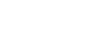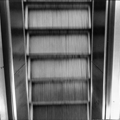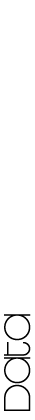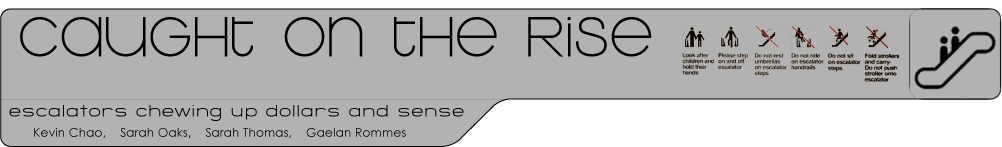See Word Document (.doc) of this File

Percent Average Occupancy:

Down escalator:

138 minutes + 1.73 minutes = 139.73 minutes/60 minutes = 2.33 hr/6 hr = 38.8%

Up escalator:

132 minutes + 3.42 minutes = 135.42 minutes/60 minutes = 2.26 hr/6 hr = 37.7%

Average of both: 38.3%

Motor Output:

Motor = 5 HP

1 HP = 746 W

(MEEB pg. 180)

5 HP x 0.746 kW = 3.73 kW

Basic Charge – Three-Phase Plan (Monthly Service Charge): \$28.25

Demand Charge = \$5.00/kW

Energy Charge = \$0.04779/kWh

(Commercial Energy Rate: http://www.eweb.org/news/projects/rates.htm)

Demand charge for motor:

3.73 kW x \$5.00 = \$18.65

Cost per hour for motor:

3.73 kW x 1 hr x \$0.04779 = \$0.178

Cooling Load: 1 W = 3.41 BTU

(Green Studio Handbook – Energy Production: Plug Loads p. 183)

3730 W x 3.41 btu = 12719.3 btu

Energy Conversion: 1 W = 3.412 btu

12719.3 btu/3.412 btu = 3730 W = 3.73 kW

3.73 kW x 1 hr x \$0.04779 = \$0.178

Energy Cost:

Energy cost per week:

Mon – Sat: 10AM – 9 PM: 11 hrs x 6 = 66 hrs

Sun – 11AM – 6PM: 7 hrs

Hours open per week = 73 hrs

73 hrs x [\$0.178 (motor) + \$0.178 (cooling)] = \$25.988 per week

Energy Use/Cost per year:

365.25/7 = 52.18 weeks per year

Use:

2(3.73 kWh) x 73 hours x 52.18 weeks = 28416.1844 kWh

Cost:

(\$25.988 x 52.18) + 12(\$28.25 + \$18.65) = \$1920.413

(weekly cost x weeks in year) + 12 months of service (basic charge + demand charge)

Cost/Energy Effectiveness:

Money utilized per hour: \$0.178 x .383 = \$0.068

Money not utilized: \$0.178 - \$0.068 = \$0.11

Money utilized:

\$1920.413 x .383 = \$735.518

Money not utilized:

\$1920.413 - \$735.518 = \$1184.895

Energy Utilized:

28416.1844 kWh x .383 = 10883.39863 kWh

Energy not utilized:

28416.1844 kWh - 10883.39863 kWh = 17532.78577 kWh